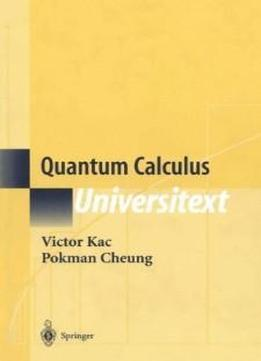# Quantum Calculus by Victor Kac / 2001 / English / PDF

Simply put, quantum calculus is ordinary calculus without taking limits. This undergraduate text develops two types of quantum calculi, the q-calculus and the h-calculus. As this book develops quantum calculus along the lines of traditional calculus, the reader discovers, with a remarkable inevitability, many important notions and results of classical mathematics. This book is written at the level of a first course in calculus and linear algebra and is aimed at undergraduate and beginning graduate students in mathematics, computer science, and physics. It is based on lectures and seminars given by MIT Professor Kac over the last few years at MIT.

Simply put, quantum calculus is ordinary calculus without taking limits. This undergraduate text develops two types of quantum calculi, the q-calculus and the h-calculus. As this book develops quantum calculus along the lines of traditional calculus, the reader discovers, with a remarkable inevitability, many important notions and results of classical mathematics. This book is written at the level of a first course in calculus and linear algebra and is aimed at undergraduate and beginning graduate students in mathematics, computer science, and physics. It is based on lectures and seminars given by MIT Professor Kac over the last few years at MIT.

views: 469